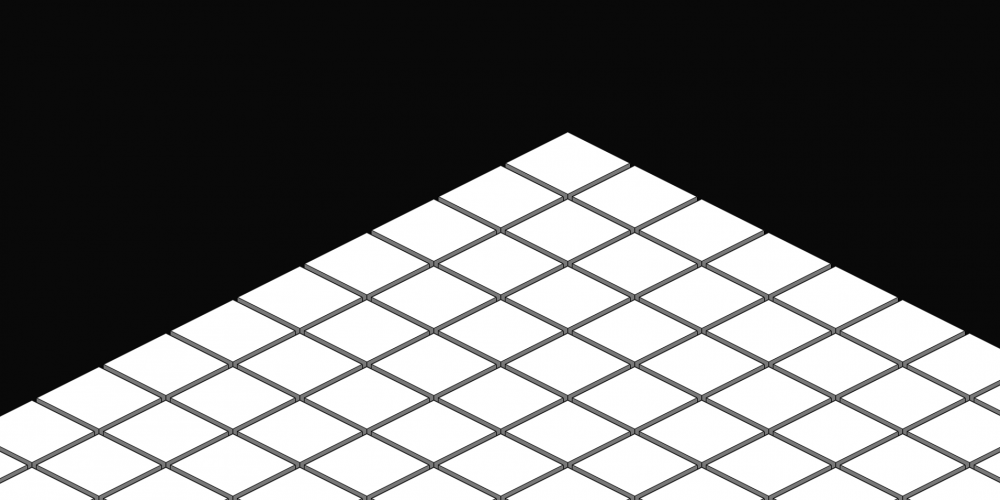# Creating an infinite grid?

## Recommended Posts

I'm creating a game that requires a grid, and while I've got the basics down, I'd like it to turn a finite grid into infinite that can be scrolled in all directions.

How would I go from this:

``````function CreateHexGrid()
{
for(var x=0; x< mapSize; ++x) {
gameMap[x] = [];
for (var y=0; y < mapSize; ++y) {
var square = new diamondTile(game, x, y);
gameMap[x][y] = square;
}
}

game.world.setBounds();
}``````

to infinite?

The image I have describes the results I have thus far.